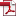• KSII Transactions on Internet and Information Systems
Monthly Online Journal (eISSN: 1976-7277)

A Novel Algorithm of Joint Probability Data Association Based on Loss Function

Vol. 15, No. 7, July 31, 202110.3837/tiis.2021.07.003, Download Paper (Free):Abstract

In this paper, a joint probabilistic data association algorithm based on loss function (LJPDA) is proposed so that the computation load and accuracy of the multi-target tracking algorithm can be guaranteed simultaneously. Firstly, data association is divided in to three cases based on the relationship among validation gates and the number of measurements in the overlapping area for validation gates. Also the contribution coefficient is employed for evaluating the contribution of a measurement to a target, and the loss function, which reflects the cost of the new proposed data association algorithm, is defined. Moreover, the equation set of optimal contribution coefficient is given by minimizing the loss function, and the optimal contribution coefficient can be attained by using the Newton-Raphson method. In this way, the weighted value of each target can be achieved, and the data association among measurements and tracks can be realized. Finally, we compare performances of LJPDA proposed and joint probabilistic data association (JPDA) algorithm via numerical simulations, and much attention is paid on real-time performance and estimation error. Theoretical analysis and experimental results reveal that the LJPDA algorithm proposed exhibits small estimation error and low computation complexity.